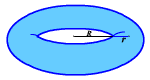# Volume

The volume of a $3$ -dimensional solid is the amount of space it occupies.  Volume is measured in cubic units ( ${\text{in}}^{3},{\text{ft}}^{3},{\text{cm}}^{3},{\text{m}}^{3}$ , et cetera).  Be sure that all of the measurements are in the same unit before computing the volume.

The following table gives the formulas for the volumes of some common solids.  Here, $r$ denotes the radius of the figure, $h$ denotes the height, $B$ denotes the area of the base, and, in the case of the torus, $R$ denotes the distance from the center of the torus to the center of the tube.

Solids
Volume
Figure
Prism

$V=Bh$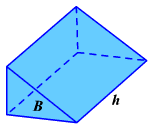Cube

$V={s}^{3}$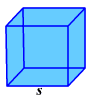Pyramid
$V=\frac{1}{3}Bh$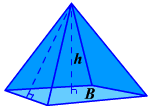Cylinder

$V=\pi {r}^{2}h$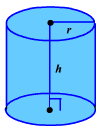Cone
$V=\frac{1}{3}\pi {r}^{2}h$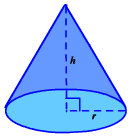Sphere
$V=\frac{4}{3}\pi {r}^{3}$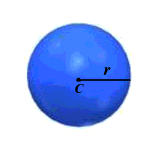Ellipsoid
$V=\frac{4}{3}\pi {r}_{1}{r}_{2}{r}_{3}$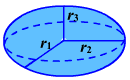Torus

$2{\pi }^{2}R{r}^{2}$